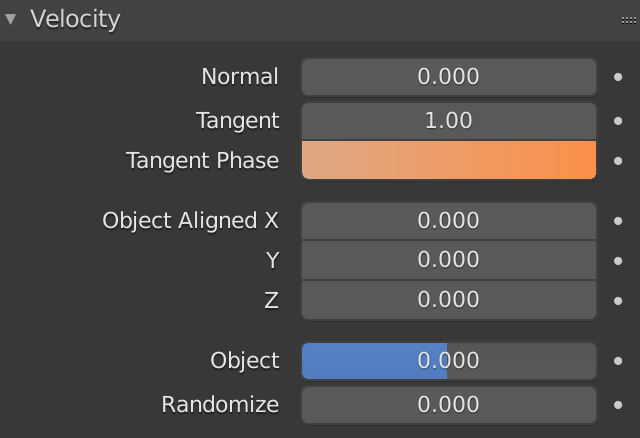# Tangent Phase

– Tangent Phase permits to modify tangent direction initially decided by blender.

– The value is expressed as percentual of 180º

– First example: 0.0 = 0º ; 0.5 = 90º

– Second example: -0.25 = -45º ; 0.25 = 45º

– Third example: 0.0 = 0º ; 1.0 = 180º